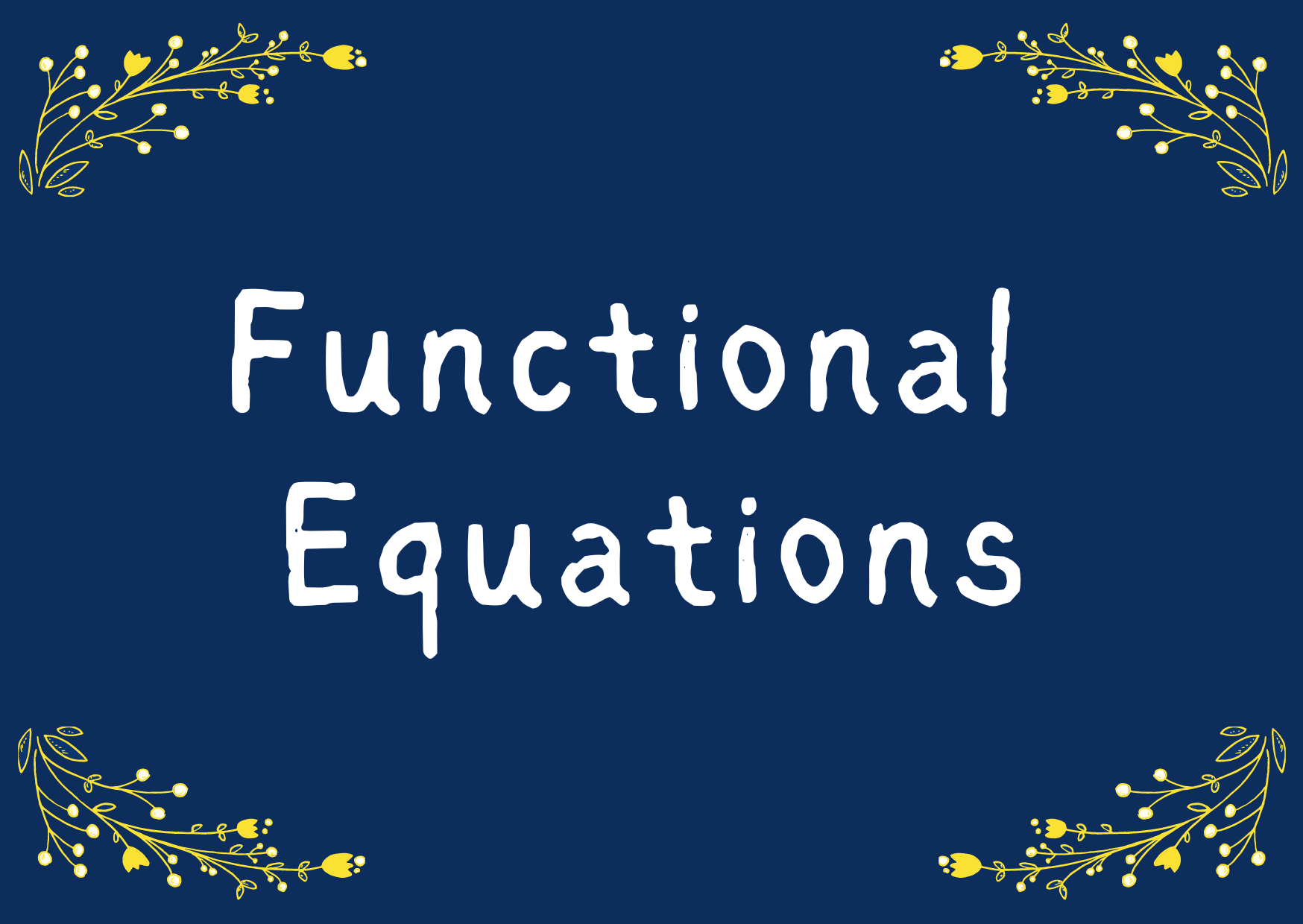# Functional Equations

1. Find all solutions f(x) of the equation xf(x) + 2xf(⎼x) = −1 where x ∈ ℝ and x ≠ 0.

2. Solve the functional equation 2f(tan x) + f(−tan x) = sin 2x, where f(x) are defined in the interval (−π/2, π/2).

3. Determine all functions f: ℝ → ℝ, such that for all x, y ∈ ℝ, (xy)f(x + y) − (x + y)f(xy) = 4xy(x2 –y2).

4. Find all polynomials p(x) satisfying the relation p(x + 1) = p(x) + 2x + 1.

5. Find all polynomials p(x) which solve the following equation for all x ∈ ℝ: xp(x − 1) = (x − 26)p(x).

6. Determine all continuous functions f: ℝ → ℝ such that f(1) = 2 and f(xy) = f(x)f(y) − f(x + y) + 1 for all x, y ∈ ℝ.

7. Find all functions f: ℝ → ℝ such that f(x) = 0 has only a finite number of roots and f(x4 + y) = x3f(x) + f(f(y)) for all x, y ∈ ℝ.

8. Determine all continuous functions f: ℝ+ → ℝ+ such that f2(x) = f(x + y)f(xy) for all x, y ∈ ℝ.

9. Find all functions f: ℕ→ ℕ which satisfy the equation f(f(f(n))) + f(f(n)) + f(n) = 3n, for all n ∈ ℕ.

10. Determine all functions f: ℝ → ℝ such that for all x ∈ ℝ and all r ∈ ℚ the inequality |f(x) − f(r)| ≤   7(xr)2 is satisfied.

11. Find all functions f: ℝ → ℝ such that f(f(x + y)) = f(x + y) + f(x)f(y) − xy for all x, y ∈ ℝ.

12. The function f(n) is defined on the positive integers ℕ and takes non-negative integer values. Moreover f(2) = 0, f(3) > 0, f(9999) = 3333 and for all m, n ∈ ℕ: f(m + n) − f(m) − f(n) = 0 or 1. Determine f(1982).

13. Determine all functions f: ℤ → ℤ such that f(−1) = f(1) and f(x) + f(y) = f(x + 2xy) + f(y − 2xy) for all x, y ∈ ℤ.

14. Find all functions f: ℝ → ℝ such that (f(x) + f(y))(f(u) + f(v)) = f(xuyv) + f(xv + yu) for all x, y, u, v ∈ ℝ.

15. Find all functions f: ℝ → ℝ such that f(x + y) − f(xy) = f(x)f(y) for all x, y ∈ ℝ.

16. Find all continuous functions f: ℝ → ℝ which satisfy the equation f(x + y) = f(x) + f(y) + xy for all x, y ∈ ℝ.

17. Find all functions f: ℝ → ℝ satisfying xf(y) + yf(x) = (x + y)f(x)f(y) for all x, y ∈ ℝ.

18. Find all injective functions f: ℝ → ℝ such that f(f(x) + y) = f(x + y) + 1 for all x, y ∈ ℝ.

19. Find all functions defined for x > 0, such that xf(y) + yf(x) = f(xy) for all x, y ∈ ℝ+.

20. Find all continuous solutions f: ℝ → ℝ to the equation f(x + y) − f(xy) = f(x) for all x, y ∈ ℝ.

1.Fauzia Rafiq Qureshi says:
1.Math1089 says: